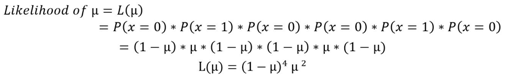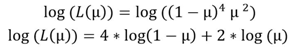# Explain maximum likelihood estimation

400    Asked by LucasBROWN in Data Science , Asked on Jan 14, 2020

Maximum likelihood estimation is a method of estimating the parameters of a model given observations, by finding the parameter values that maximize the likelihood of making the observations, this means finding parameters that maximize the probability p of event 1 and (1-p) of non-event 0, as we know:

probability (event + non-event) = 1

Let a sample (0, 1, 0, 0, 1, 0) be drawn from binomial distribution. Now let us calculate the maximum likelihood estimate of μ.

Given the fact that for binomial distribution P(X=1) = μ and P(X=0) = 1- μ where μ is the parameter:Since maximizing likelihood is the same as the maximizing log of likelihood so, log is applied to both sides of the equation for mathematical convenienceDetermining the maximum value of μ by equating derivative to zero:Hence it has been proven that at value μ = 1/3, it is maximizing the likelihood.